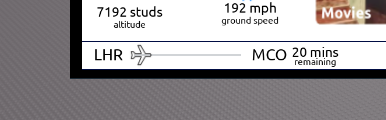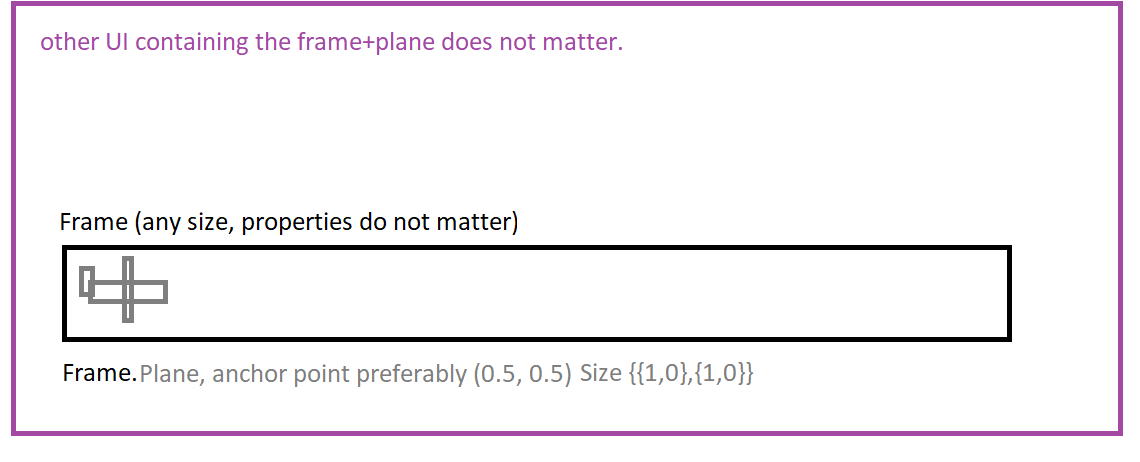# Progress bar between part A and part B calucation

I’m making an IFE for an airline and one of the features I wanted to add is a progress bar on how close the airplane is to the destination.

The issue I’m having is the position of the plane on the UI. I have got the percentage part right which ranges from 0 to 1. The thing I don’t know how to do now is to calculate the position on the UI.

I have tried to get the Positions of both UIs and find the magnitude between the UIs.

``````local DistanceBetween = (DestinationAriport.Position - StartingAirport.Position).Magnitude

function PLanePositionLogic()
local PlayerDistance = (Player.Character.HumanoidRootPart.Position - StartingAirport.Position).Magnitude
local Percentage = math.clamp((PlayerDistance / DistanceBetween),0,1)
PlaneIcon.Position = UDim2.new(Percentage / DistanceBetweenUI ,0,0,0)

end
``````If this is within a frame then the X Scale is already on a 0-1 scale. You would only have to do `UDim2.new(Percentage,0,0,0)`

The X scale is not 0-1 though. The end X Value is 0.257 and the starting X value is 0.095

You can use lerp if you want to. Lerping is very common and using a variable called “t” (a 0-1 var) you can choose between 2 values.

Formula: t*(b-a)+a

Implementation: Percentage*(0.257-0.095)+0.095

2 Likes

Absolute genius, this worked perfectly! Thank you so much.

1 Like

The X scale is 0-1, you are looking at absolute values which do not matter as the UI will automatically translate the UDim Scale into what ever size is needed.In this sample if you set `Frame.Plane.Position = UDim2.new(percent,0,0.5,0)` you will get the results you want.

1 Like